Home > MC2 > Chapter 11 > Lesson 11.2.2 > Problem11-44

11-44.
1. Which table or tables below show a proportional relationship? Justify your answers. Homework Help ✎

1.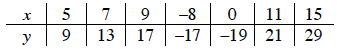2.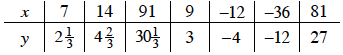3.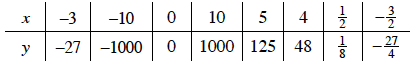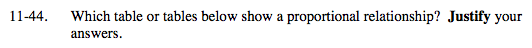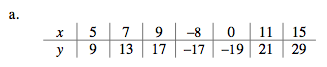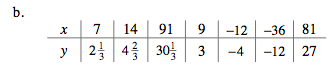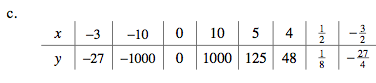A proportional equation can be written using an equation of the form y = kx.

Based on the equation above, a relationship that is proportional must contain the point (0,0) and have a constant rate of growth represented by a constant, k.

Which of these tables has these attributes?

Rearrange the ordered pairs so the x-values are listed from smallest to largest x-values.

Table (b) could contain the point (0,0) and grows at a constant rate.